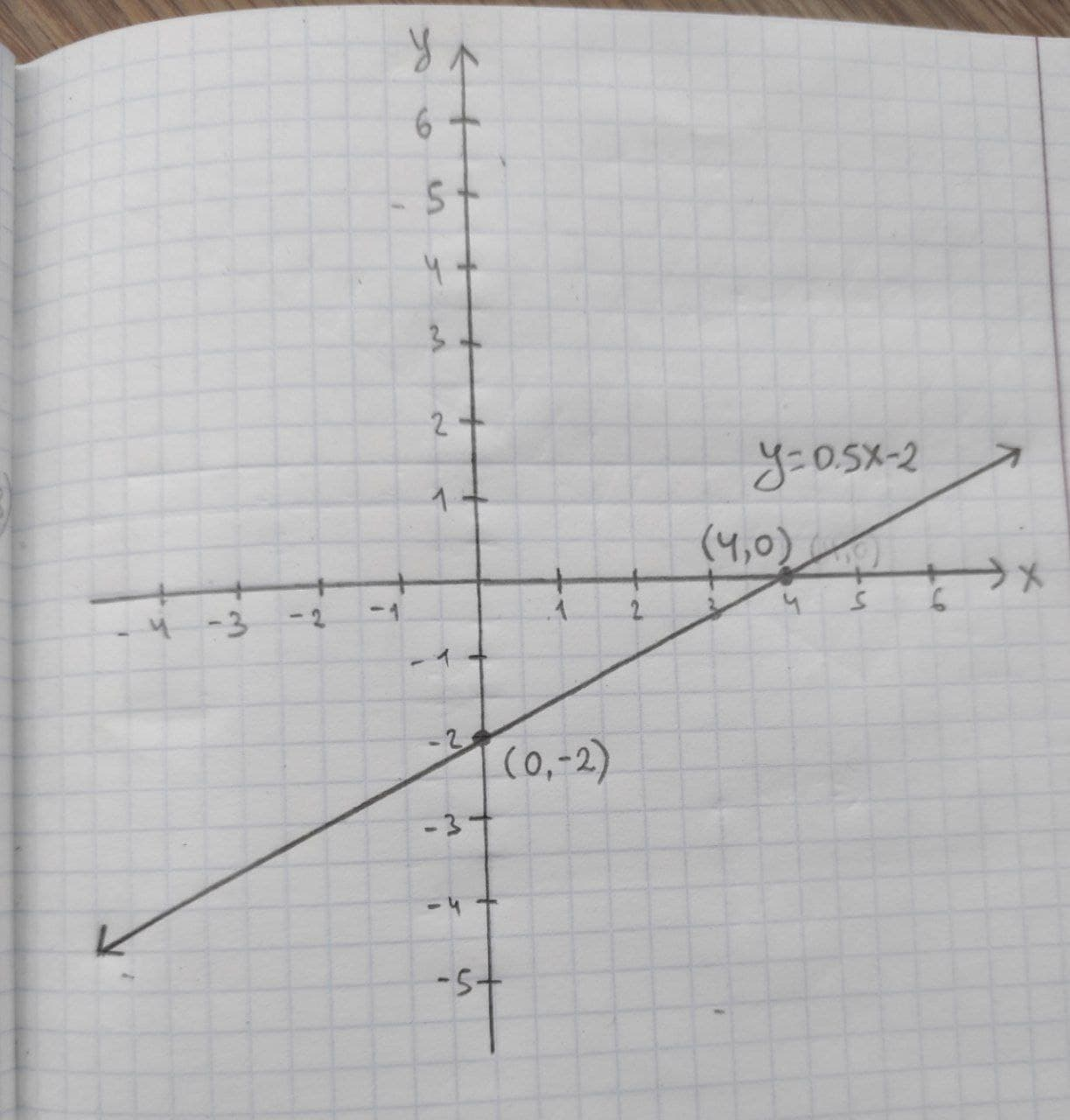# Given linear equation y = 0.5x – 2 a. find the y-intercept and slope. b. determine whether the line slopes upward, slopes downward, or is horizontal, without graphing the equation. c. use two points to graph the equation.necessaryh 2020-11-05 Answered
Given linear equation y = 0.5x – 2
a. find the y-intercept and slope.
b. determine whether the line slopes upward, slopes downward, or is horizontal, without graphing the equation.
c. use two points to graph the equation.

• Questions are typically answered in as fast as 30 minutes

### Plainmath recommends

• Get a detailed answer even on the hardest topics.
• Ask an expert for a step-by-step guidance to learn to do it yourself.casincal

(a) y-intercept and slope: In a linear equation $$y=b_0+b_1x$$, the constant $$b1$$ be the slope and $$b_0$$ be the y-intercept form and x is the independent variable and y is the dependent variable. Comparing the given equation with the general form of linear equation the slope of the equation is 0.5 and the y-intercept is –2. Therefore, the y-intercept is –2 and the slope of the linear equation (b_1) is 0.5. (b) It is known that, the slope of the linear equation $$\displaystyle{y}={b}_{{0}}+{b}_{{1}}{x}$$ is upward if b_1 > 0, the slope of the linear equation $$\displaystyle{y}={b}_{{0}}+{b}_{{1}}{x}$$ is downward if $$\displaystyle{b}_{{1}}{<}{0}$$, and the slope of the linear equation $$\displaystyle{y}={b}_{{0}}+{b}_{{1}}{x}$$ is horizontal if $$\displaystyle{b}_{{1}}={0}$$ .
Thus, in the given equation $$\displaystyle{y}={0.5}{x}–{2},{b}_{{1}}$$ is 0.5, which is greater than 0.
Thus, the slope is upward.
(c) Graph by using two points:
The two points $$\displaystyle{\left({x}_{{1}},{y}_{{1}}\right)}{\quad\text{and}\quad}{\left({x}_{{2}},{y}_{{2}}\right)}$$ on the given line are obtained as follows:
If x=0
$$\displaystyle{y}={\left({0},{5}\times{0}\right)}-{2}$$
y=-2
Thus, one point on the line is $$\displaystyle{\left({x}_{{1}},{y}_{{1}}\right)}={\left({0},-{2}\right)}$$
If x=4,
$$\displaystyle{y}={\left({0.5}\times{4}\right)}-{2}$$
y=0
Thus, the second point on the line is $$\displaystyle{\left({x}_{{2}},{y}_{{2}}\right)}={\left({4},{0}\right)}$$